# Analytical Plotting with Symbolic Math Toolbox

Symbolic Math Toolbox™ provides analytical plotting of mathematical expressions without explicitly generating numerical data. These plots can be in 2-D or 3-D as lines, curves, contours, surfaces, or meshes.

These examples feature the following graphics functions that accept symbolic functions, expressions, and equations as inputs:

• fplot

• fimplicit

• fcontour

• fplot3

• fsurf

• fmesh

• fimplicit3

### Plot Explicit Functions $y=f\left(x\right)$ Using fplot

Plot the function $\mathrm{sin}\left(\mathrm{exp}\left(x\right)\right)$.

syms x fplot(sin(exp(x)))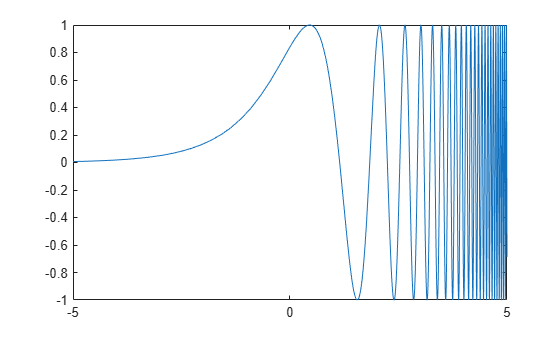Plot the trigonometric functions $\mathrm{sin}\left(x\right)$, $\mathrm{cos}\left(x\right)$, and $\mathrm{tan}\left(x\right)$ simultaneously.

fplot([sin(x),cos(x),tan(x)])### Plot a Function Defined by $y=f\left(x,a\right)$ for Various Values of $a$

Plot the function $\mathrm{sin}\left(\mathrm{exp}\left(x/a\right)\right)$ for $a=1,2$, and $4$.

syms x a expr = sin(exp(x/a)); fplot(subs(expr,a,[1,2,4])) legend show### Plot the Derivative and Integral of a Function

Plot a function $f\left(x\right)=x\phantom{\rule{0.2222222222222222em}{0ex}}\left(1+x\right)+2$, its derivative $df\left(x\right)/dx$, and its integral $\int f\left(x\right)\phantom{\rule{0.2777777777777778em}{0ex}}dx$.

syms f(x) f(x) = x*(1 + x) + 2
f(x) = $x \left(x+1\right)+2$
f_diff = diff(f(x),x)
f_diff = $2 x+1$
f_int = int(f(x),x)
f_int =  $\frac{x \left(2 {x}^{2}+3 x+12\right)}{6}$
fplot([f,f_diff,f_int]) legend({'$f(x)$','$df(x)/dx$','$\int f(x)dx$'},'Interpreter','latex','FontSize',12)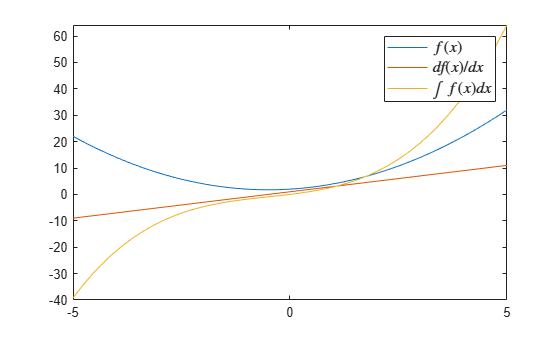### Plot a Function $y=g\left({x}_{0},a\right)$ with $a$ as the Horizontal Axis

Find the ${x}_{0}$ that minimizes a function $g\left(x,a\right)$ by solving the differential equation $dg\left(x,a\right)/dx=0$.

syms g(x,a); assume(a>0); g(x,a) = a*x*(a + x) + 2*sqrt(a)
g(x, a) = $2 \sqrt{a}+a x \left(a+x\right)$
x0 = solve(diff(g,x),x)
x0 =  $-\frac{a}{2}$

Plot the minimum value of $g\left({x}_{0},a\right)$ for $a$ from 0 to 5.

fplot(g(x0,a),[0 5]) xlabel('a') title('Minimum Value of $g(x_0,a)$ Depending on $a$','interpreter','latex')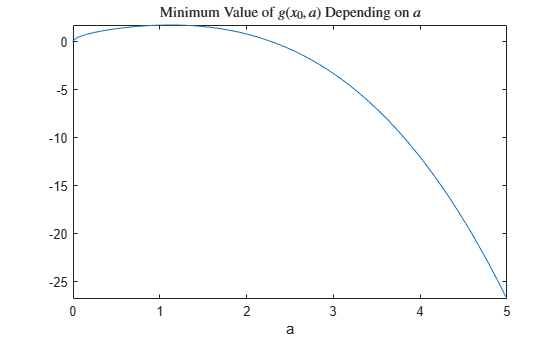### Plot an Implicit Function $f\left(x,y\right)=c$ Using fimplicit

Plot circles defined by ${x}^{2}+{y}^{2}={r}^{2}$ with radius $r$ as the integers from 1 to 10.

syms x y r = 1:10; fimplicit(x^2 + y^2 == r.^2,[-10 10]) axis square;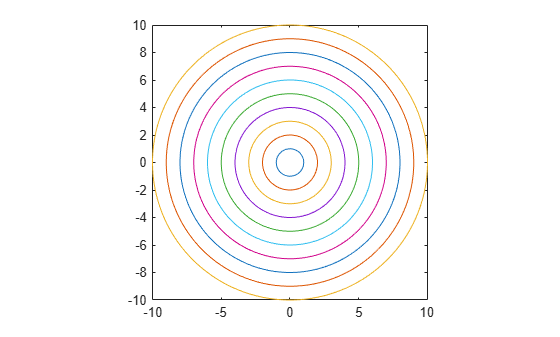### Plot Contours of a Function $f\left(x,y\right)$ Using fcontour

Plot contours of the function $f\left(x,y\right)={x}^{3}-4x-{y}^{2}$ for contour levels from –6 to 6.

syms x y f(x,y) f(x,y) = x^3 - 4*x - y^2; fcontour(f,[-3 3 -4 4],'LevelList',-6:6); colorbar title 'Contour of Some Elliptic Curves'### Plot an Analytic Function and Its Approximation Using Spline Interpolant

Plot the analytic function $f\left(x\right)=x\phantom{\rule{0.2222222222222222em}{0ex}}\mathrm{exp}\left(-x\right)\mathrm{sin}\left(5x\right)-2$.

syms f(x) f(x) = x*exp(-x)*sin(5*x) -2; fplot(f,[0,3])

Create a few data points from the analytic function.

xs = 0:1/3:3; ys = double(subs(f,xs));

Plot the data points and the spline interpolant that approximates the analytic function.

hold on plot(xs,ys,'*k','DisplayName','Data Points') fplot(@(x) spline(xs,ys,x),[0 3],'DisplayName','Spline interpolant') grid on legend show hold off### Plot Taylor Approximations of a Function

Find the Taylor expansion of $\mathrm{cos}\left(x\right)$ near $x=0$ up to 5th and 7th orders.

syms x t5 = taylor(cos(x),x,'Order',5)
t5 =  $\frac{{x}^{4}}{24}-\frac{{x}^{2}}{2}+1$
t7 = taylor(cos(x),x,'Order',7)
t7 =  $-\frac{{x}^{6}}{720}+\frac{{x}^{4}}{24}-\frac{{x}^{2}}{2}+1$

Plot $\mathrm{cos}\left(x\right)$ and its Taylor approximations.

fplot(cos(x)) hold on; fplot([t5 t7],'--') axis([-4 4 -1.5 1.5]) title('Taylor Series Approximations of cos(x) up to 5th and 7th Order') legend show hold off;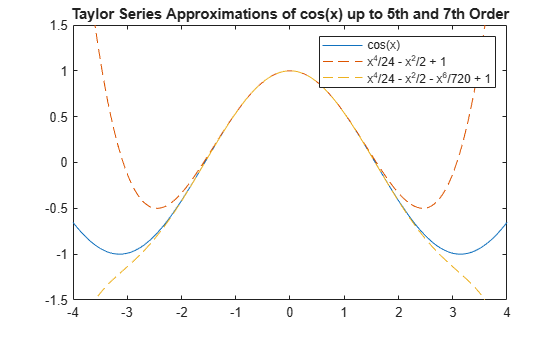### Plot the Fourier Series Approximation of a Square Wave

A square wave of period $2\pi$ and amplitude $\pi /4$ can be approximated by the Fourier series expansion

$\mathrm{sin}\left(t\right)+\frac{1}{3}\mathrm{sin}\left(3t\right)+\frac{1}{5}\mathrm{sin}\left(5t\right)+...\phantom{\rule{0.2777777777777778em}{0ex}}.$

Plot a square wave with period $2\pi$ and amplitude $\pi /4$.

syms t y(t) y(t) = piecewise(0 < mod(t,2*pi) <= pi, pi/4, pi < mod(t,2*pi) <= 2*pi, -pi/4); fplot(y)

Plot the Fourier series approximation of the square wave.

hold on; n = 6; yFourier = cumsum(sin((1:2:2*n-1)*t)./(1:2:2*n-1)); fplot(yFourier,'LineWidth',1) hold offThe Fourier series approximation overshoots at a jump discontinuity and the "ringing" does not die out as more terms are added to the approximation. This behavior is also known as the Gibbs phenomenon.

### Plot a Parametric Curve $\left(x\left(t\right),y\left(t\right),z\left(t\right)\right)$ Using fplot3

Plot a helix that is defined by $\left(\mathrm{sin}\left(t\right),\phantom{\rule{0.2777777777777778em}{0ex}}\mathrm{cos}\left(t\right),\phantom{\rule{0.2777777777777778em}{0ex}}t/4\right)$ for $t$ from –10 to 10.

syms t fplot3(sin(t),cos(t),t/4,[-10 10],'LineWidth',2) view([-45 45])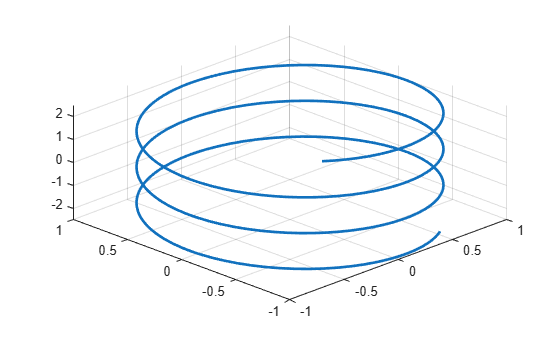### Plot a Surface Defined by $z=f\left(x,y\right)$ Using fsurf

Plot a surface defined by $\mathrm{log}\left(x\right)+\mathrm{exp}\left(y\right)$. Analytical plotting using fsurf (without generating numerical data) shows the curved areas and asymptotic regions near $x=0$.

syms x y fsurf(log(x) + exp(y),[0 2 -1 3]) xlabel('x')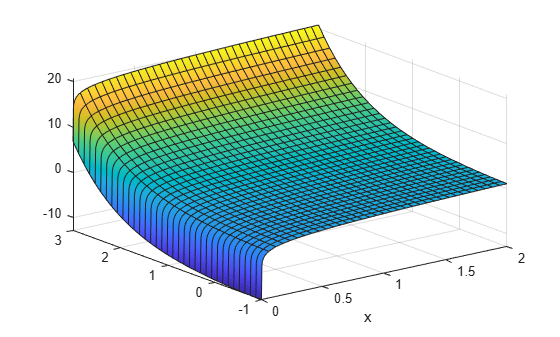### Plot a Multivariate Surface $\left(x\left(u,v\right),\phantom{\rule{0.2777777777777778em}{0ex}}y\left(u,v\right),\phantom{\rule{0.2777777777777778em}{0ex}}z\left(u,v\right)\right)$ Using fsurf

Plot a multivariate surface defined by

$x\left(u,v\right)=u$

$y\left(u,v\right)=f\left(u\right)\phantom{\rule{0.2777777777777778em}{0ex}}\mathrm{sin}\left(v\right)$

$z\left(u,v\right)=f\left(u\right)\phantom{\rule{0.2777777777777778em}{0ex}}\mathrm{cos}\left(v\right)$

where $f\left(u\right)=\mathrm{exp}\left(-{u}^{2}/3\right)\mathrm{sin}\left(u\right)+3/2$.

Set the plot interval of $u$ from –5 to 5 and $v$ from 0 to 2$\pi$.

syms f(u) x(u,v) y(u,v) z(u,v) f(u) = sin(u)*exp(-u^2/3)+1.5; x(u,v) = u; y(u,v) = f(u)*sin(v); z(u,v) = f(u)*cos(v); fsurf(x,y,z,[-5 5 0 2*pi])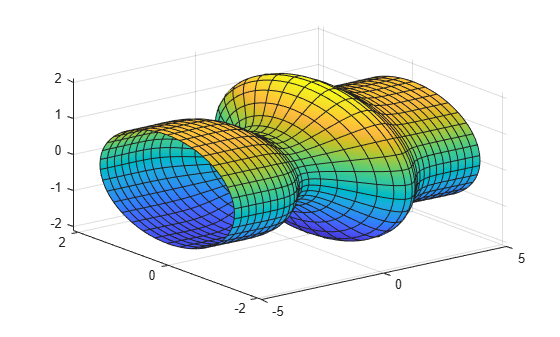### Plot a Multivariate Surface $\left(x\left(s,t\right),\phantom{\rule{0.2777777777777778em}{0ex}}y\left(s,t\right),\phantom{\rule{0.2777777777777778em}{0ex}}z\left(s,t\right)\right)$ Using fmesh

Plot a multivariate surface defined by

$x=r\phantom{\rule{0.2222222222222222em}{0ex}}\mathrm{cos}\left(s\right)\phantom{\rule{0.2222222222222222em}{0ex}}\mathrm{sin}\left(t\right)$

$y=r\phantom{\rule{0.2222222222222222em}{0ex}}\mathrm{sin}\left(s\right)\phantom{\rule{0.2222222222222222em}{0ex}}\mathrm{sin}\left(t\right)$

$z=r\phantom{\rule{0.2777777777777778em}{0ex}}\mathrm{cos}\left(t\right)$

where $r=8+\mathrm{sin}\left(7s+5t\right)$. Show the plotted surface as meshes by using fmesh. Set the plot interval of $s$ from 0 to 2$\pi$ and $t$ from 0 to $\pi$.

syms s t r = 8 + sin(7*s + 5*t); x = r*cos(s)*sin(t); y = r*sin(s)*sin(t); z = r*cos(t); fmesh(x,y,z,[0 2*pi 0 pi],'Linewidth',2) axis equal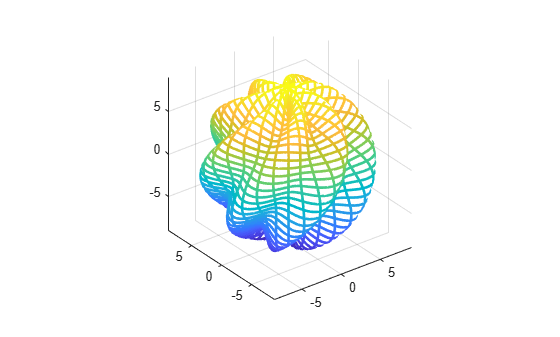### Plot an Implicit Surface $f\left(x,y,z\right)=c$ Using fimplicit3

Plot the implicit surface $1/{x}^{2}-1/{y}^{2}+1/{z}^{2}=0$.

syms x y z f = 1/x^2 - 1/y^2 + 1/z^2; fimplicit3(f)### Plot the Contours and Gradient of a Surface

Plot the surface $\mathrm{sin}\left(x\right)+\mathrm{sin}\left(y\right)-\left({x}^{2}+{y}^{2}\right)/20$ using fsurf. You can show the contours on the same graph by setting 'ShowContours' to 'on'.

syms x y f = sin(x)+sin(y)-(x^2+y^2)/20
f =  $\mathrm{sin}\left(x\right)+\mathrm{sin}\left(y\right)-\frac{{x}^{2}}{20}-\frac{{y}^{2}}{20}$
fsurf(f,'ShowContours','on') view(-19,56)Next, plot the contours on a separate graph with finer contour lines.

fcontour(f,[-5 5 -5 5],'LevelStep',0.1,'Fill','on') colorbar

Find the gradient of the surface. Create 2-D grids using meshgrid and substitute the grid coordinates to evaluate the gradient numerically. Show the gradient using quiver.

hold on Fgrad = gradient(f,[x,y])
Fgrad =  $\left(\begin{array}{c}\mathrm{cos}\left(x\right)-\frac{x}{10}\\ \mathrm{cos}\left(y\right)-\frac{y}{10}\end{array}\right)$
[xgrid,ygrid] = meshgrid(-5:5,-5:5); Fx = subs(Fgrad(1),{x,y},{xgrid,ygrid}); Fy = subs(Fgrad(2),{x,y},{xgrid,ygrid}); quiver(xgrid,ygrid,Fx,Fy,'k') hold off